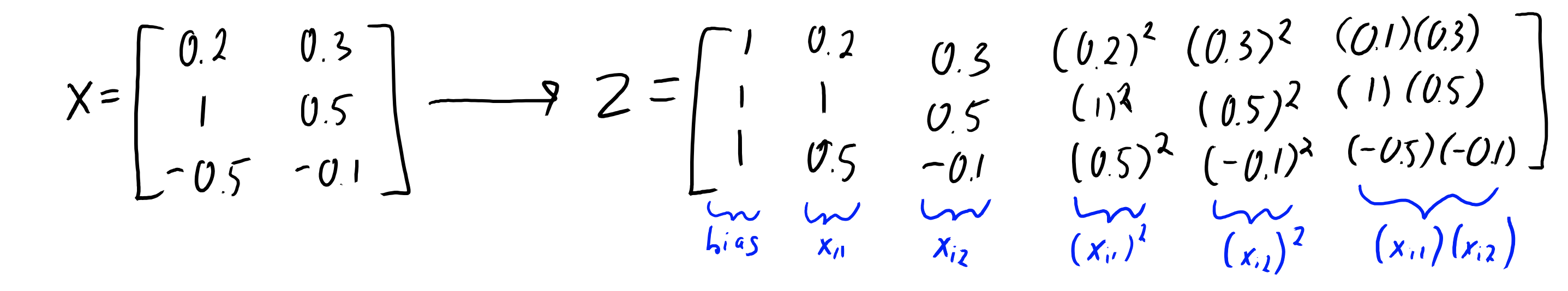Search

# Change of basis

Last updated Nov 3, 2022 Edit Source

Effectively by constructing new features that take the variable to certain powers. To get a y-intercept (bias), we just raise $x$ to the 0th power to get 1. We can fit polynomials of degree $p$ by raising other powers:

$$Z = \begin{bmatrix} 1 & x_1 & x_1^2 & \dots & x_1^p \\ 1 & x_2 & x_2^2 & \dots & x_2^p \\ \vdots & \vdots & \vdots & \ddots & \vdots \\ 1 & x_n & x_n^2 & \dots & x_n^p \end{bmatrix}$$

As the polynomial degree increases, the training error goes down but the approximation error goes up.

Choosing a basis is hard! We can do something like Gaussian radial basis functions (RBFs) or polynomial basis as these are both universal approximators given enough data.

## # Kernel Trick

Let $Z$ be the basis. With multi-dimensional polynomial bases, actually forming $Z$ which is $k = O(d^p)$ is intractable.

Represent each column of $Z$ as a unique term. For example, with an $X$ of $d=2$, we can use $p=2$ to getWe compute $u = (K + \lambda I)^{-1}y$

and for testing:

\begin{aligned} \hat y &= \tilde Z v \\ &= \tilde Z Z^T (ZZ^T + \lambda I)^{-1} y & \textrm{minimum of L2-regularized least squares: } v = Z^T(ZZ^T + \lambda I)^{-1}y \\ &= \tilde K (K + \lambda I)^{-1} y \\ &= \tilde K u & u \textrm{ is a (n,1) of kernel weights we learn} \end{aligned}

The key idea behind “kernel trick” for certain bases (like polynomials) is that we can efficiently compute $K$ and $\tilde K$ even though forming $Z$ and $\tilde Z$ is intractable.

We call $K = ZZ^T$ the Gram Matrix.

Finally, we call the general degree-p polynomial kernel function $K_{ij} = k(x_i, x_j) = (1 + x_i^Tx_j)^p$. Computing $k$ is only $O(d)$ time instead of $O(d^p)$.

Thus, computing $K$ is $O(n^2d + n^3)$:

1. Forming $K$ takes $O(n^2d)$ time
2. Inverting $K+\lambda I$ which is a $(n,n)$ takes $O(n^3)$

All of our distance-based methods have kernel versions

## # Learned Basis

We can also learn basis from data as well. See latent-factor model